Next: Kinematics types Up: Various identifiers Previous: The S Reaction Switch   Contents

# The I_number identifier of the kinds of data

The reaction data identifier, I_number, tells what data is stored, as given by the following table.

0:
This type of data contains pairs (energy, cross section). For incident photons mcfgen does log-log interpolation onto a fixed set of energy points, and for other incident particles it computes averages over energy groups as described in Section 1.5.3.

1:
The data consists of pairs (cosine of the collision angle, probability density) for a sequence of incident energies. This data may be in the center-of-mass system or the laboratory system, depending on the kinematics type of the reaction. The code mcfgen converts this data into equiprobable bins.

3:
The data consists of pairs (energy of secondary particle, probability density) for a sequence of incident energies and cosines of the collision angle. This data is in the center-of-mass system. The probability over cosine and energy of the secondary is correlated, but the data is not normalized 2-dimensionally. Instead, the integral of the probability density over the energy of the secondary particle is itself 1. Consequently, the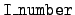= 3 data must be supplemented by= 1 data giving the angular distributions. The output is a collection of equiprobable 2-dimensional (cosine, energy) bins.

4:
The data consists of pairs (energy of secondary particle, probability density) for a sequence of incident energies. This data is in the laboratory system. The output of mcfgen in this case is equiprobable energy bins.

7:
The data consists of pairs (incident energy, multiplicity) for fission neutrons. These neutrons may be prompt or delayed. The code mcfgen computes group averages of this data, as described in Section 1.5.3.

9:
The data consists of pairs (incident energy, multiplicity) for emitted photons. The treatment of this data is the same as for= 7.

10:
The data consists of pairs (incident energy, energy of secondary particle). This data may be in the center-of-mass system or the laboratory system, depending on the kinematics type of the reaction. For incident photons mcfgen does linear-linear interpolation onto a fixed set of energy points, and for other incident particles it computes averages over energy groups as described in Section 1.5.3.

11:
The data consists of pairs (incident energy, energy deposited to the residual nucleus). This data is not used by mcfgen.

12:
The data consists of pairs (incident energy, Q), where Q denotes the sum of the energies available from the non-elastic reactions. Group averages of this data are calculated as described in Section 1.5.3.

941:
Coherent scattering form factors for incident photons. The coherent scattering form factor FR(E) specifies the importance of Rayleigh scattering. Specifically, for Rayleigh scattering from a target with atomic number Z the cross section is C(Z)(1 +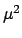), where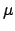is the cosine of the collision angle and C(Z) is a coefficient depending on the target. Then [1, p. 3-23] the angular distribution for coherent scattering for such a target is given by the formula

f (E,) = [FR(E)]2C(Z)(1 +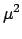).

The data consists of pairs of grid-based data (incident energy, form factor), and this information is printed in the mcf.asc' output file.

942:
Incoherent scattering form factors for incident photons. The incoherent scattering form factor FC(E) specifies the importance of Compton scattering. Specifically, the Klein-Nishina formula for the Compton scattering cross section is

KN(E,) =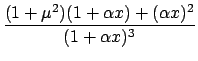,

where E is the incident energy,is the cosine of the collision angle,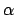is the photon energy in units of electron rest energy, and x = 1 -. The angular distribution for incoherent scattering is given by [1, p. 3-23]

f (E,) = FC(E)KN(E,).

The data consists of pairs of grid-based data (incident energy, form factor), and this information is printed in the mcf.asc' output file. Incidentally, the energy of the secondary photon (in units of electron rest energy,) is given by/(1 +x).Next: Kinematics types Up: Various identifiers Previous: The S Reaction Switch   Contents